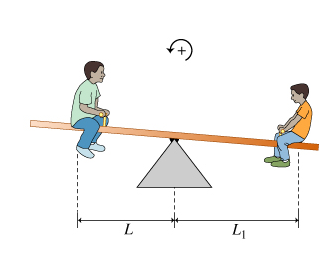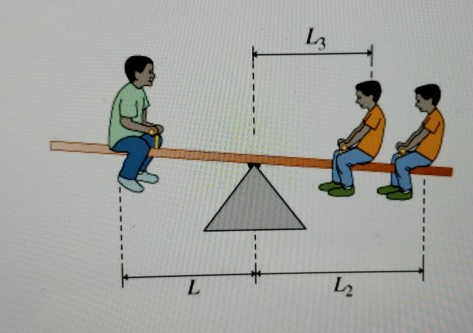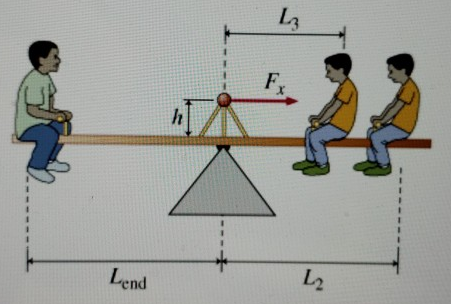###### FREE Expert Solution

Torque:

$\overline{){\mathbit{\tau }}{\mathbf{=}}{\mathbit{r}}{\mathbit{F}}}$

Rotational equilibrium:

$\overline{){\mathbf{\Sigma }}{\mathbf{\tau }}{\mathbf{=}}{\mathbf{0}}}$

Part A

Counterclockwise is positive.

81% (372 ratings)###### Problem DetailsLearning Goal: To make the connection between intuitive understanding of a seesaw and the standard formalism for torque. This problem deals with the concept of torque, the "twist" that an off-center force applies to a body that tends to make it rotate. (Figure 1)

Marcel wants the seesaw to balance, which means that there can be no angular acceleration about the pivot. For the angular acceleration to be zero, the sum of the torques about the pivot must equal zero:

Part A

Determine the sum of the torques on the seesaw. Consider only the torques exerted by the children.

Gilles has an identical twin, Jean, also of weight w. The two twins now sit on the same side of the seesaw, with Gilles at distance L2 from the pivot and Jean at distance L3. (Figure 2)Part B

Where should Marcel position Jacques to balance the seesaw?

Bad news! When Marcel finds the distance L from the previous part, it turns out to be greater than Lend, the distance from the pivot to the end of the seesaw. Hence, even with Jacques at the very end of the seesaw, the twins Gilles and Jean exert more torque than Jacques does. Marcel now elects to balance the seesaw by pushing sideways on an ornament (shown in red) that is at height above the pivot. (Figure 3)Part C

With what force in the rightward direction, Fx, should Marcel push? If your expression would give a negative result (using actual values) that just means the force should be toward the left.

Express your answer in terms of W, Lend, w, L2, L3, and h.